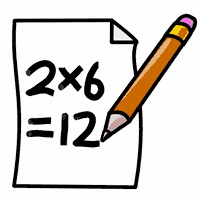Definition of

# CalculateTo work out an answer, usually by adding, multiplying etc.

Example: Calculate the cost of 10 apples when each apple costs 0.50.
Answer: 10 x 0.50 = 5.00